# Samacheer Kalvi Books: Tamilnadu State Board Text Books Solutions

## Samacheer Kalvi 11th Computer Science Chapter 11 Functions Notes PDF Download: Tamil Nadu STD 11th Computer Science Chapter 11 Functions NotesSamacheer Kalvi 11th Computer Science Chapter 11 Functions Notes PDF Download: Tamil Nadu STD 11th Computer Science Chapter 11 Functions Notes

## Samacheer Kalvi 11th Computer Science Chapter 11 Functions Notes PDF Download

We bring to you specially curated Samacheer Kalvi 11th Computer Science Chapter 11 Functions Notes PDF which have been prepared by our subject experts after carefully following the trend of the exam in the last few years. The notes will not only serve for revision purposes, but also will have several cuts and easy methods to go about a difficult problem.

 Board Tamilnadu Board Study Material Notes Class Samacheer Kalvi 11th Computer Science Subject 11th Computer Science Chapter Chapter 11 Functions Format PDF Provider Samacheer Kalvi Books

## How to Download Samacheer Kalvi 11th Computer Science Chapter 11 Functions Notes PDFs?

2. Click on the Samacheer Kalvi 11th Computer Science Notes PDF.
3. Look for your preferred subject.
4. Now download the Samacheer Kalvi 11th Computer Science Chapter 11 Functions notes PDF.

## Download Samacheer Kalvi 11th Computer Science Chapter 11 Functions Chapterwise Notes PDF

PART – 1

Question 1.
Which of the following header file defines the standard I/O predefined functions?
(a) stdio.h
(b) math.h
(c) string.h
(d) ctype.h
(a) stdio.h

Question 2.
Which function is used to check whether a character is alphanumeric or not?
(a) isalpha()
(b) isdigit()
(c) isalnum()
(d) islower()
(c) isalnum()

Question 3.
Which function begins the program execution?
(a) isalpha()
(b) isdigit()
(c) main()
(d) islower()
(c) main()

Question 4.
Which of the following function is with a return value and without any argument?
(a) x = display(int, int)
(b) x = display()
(c) y = display(float)
(d) display(int)
(b) x = display()

Question 5.
Which is return data type of the function prototype of add(int, int);?
(a) int
(b) float
(c) char
(d) double
(a) int

Question 6.
Which of the following is the scope operator?
(a) >
(b) &
(c) %
(d) ::
(d) ::

PART – 2
II. Answer to all the questions

Question 1.
Define Functions.
A large program can typically be split into smaller sized blocks called as functions.
Functions can be classified into Pre-defined or Built-in or Library Functions and User-defined Functions.

Question 2.
The strlen() takes a null-terminated byte string source as its argument and returns its length. The length does not include the null(\0) character.

Question 3.
What are the importance of void data type?
void type has two important purposes:

1. To indicate the function does not return a value
2. To declare a generic pointer.

Question 4.
What are Parameter and list its types?
Arguments or parameters are the means to pass values from the calling function to the called function. The variables used in the function definition as parameters are known as formal parameters. The constants, variables, or expressions used in the function call are known as actual parameters.
Types: Default arguments and Constant Arguments.

Question 5.
Write a note on Local Scope.

1. A local variable is defined within a block.A block of code begins and ends with curly braces { }.
2. The scope of a local variable is the block in which it is defined.
3. A local variable cannot be accessed from outside the block of its declaration.
4. A local variable is created upon entry into its block and destroyed upon exit.

PART – 3
III. Answer to all the questions

Question 1.
What is Built-in functions?
C++ provides a rich collection of functions ready to be used for various tasks. The tasks to be performed by each of these are already written, debugged and compiled, their definitions alone are grouped and stored in files called header files. Such ready – to – use subprograms are called predefined functions or built-in functions.

Question 2.
What is the difference between isupper() and toupper() functions?
isupper( )
This function is used to check the given character is uppercase. This function will return 1 if true otherwise 0. For the following examples value 1 will be assigned to n and 0 for m.
int n=isupper(‘A’);
int m=isupper(‘a’);

toupper( )
This function is used to convert the given character into its uppercase. This function will return the upper case equivalent of the given character. If the given character itself is in upper case, the output will be the same.
The following statement will assign the character constant ‘K’ to the variable c.

char c = toupper(‘k’);
But, the output of the statement given below will be ‘B’ itself.
cout <<toupper(‘B’);

Question 3.
The strcmp() function takes two arguments: string1 and string2. It compares the contents of string1 and string2 lexicographically.
The strcmp() function returns a:

1. Positive value if the first differing character in string1 is greater than the corresponding character in string2. (ASCII values are compared)
2. Negative value if the first differing character in string1 is less than the corresponding character in string2.
3. 0 if string1 and string2 are equal.

Question 4.
Write short note on pow() function in C++.
The pow() function returns base raised to the power of the exponent. If any argument passed to pow() is long double, the return type is promoted to long double. If not, the return type is double. The pow() function takes two arguments:

1. base – the base value
2. exponent – exponent of the base

Example:
cout << pow(5, 2);

Output:
25

Question 5.
What are the information the prototype provides to the compiler?
The function prototype provides details about the return data type, name of the function and a list of formal parameters or arguments.

Consider the following function prototype:
long fact (int, double);

The prototype above provides the following information to the compiler:

• The return value of the function is of type long.
• fact is the name of the function.
• The function is called with two arguments:
• The first argument is of int data type.
• The second argument is of double data type.

Question 6.
What is default arguments? Give example.
In C++, one can assign default values to the formal parameters of a function prototype. The Default arguments allow omitting some arguments when calling the function.
1. For any missing arguments, compiler uses the values in default arguments for the called function.

2. The default value is given in the form of variable initialization.
Example : void defaultvalue(int n1 = 10, n2 = 100);

3. The default arguments facilitate the function call statement with partial or no arguments.
Example :

1. defaultvalue (x, y);
2. defaultvalue (200, 150);
3. defaultvalue (150);
4. defaultvalue (x, 150);

4. The default values can be included in the function prototype from right to left, i.e., we cannot have a default value for an argument in between the argument list.
Example:

1. void defaultvalue (int n1=10, n2);//invalid prototype.
2. void defaultvalue (int n1, n2 = 10);//valid prototype.

PART – 4
IV. Answers to all the questions

Question 1.
Explain Call by value method with suitable example.
This method copies the value of an actual parameter into the formal parameter of the function. In this case, changes made to formal parameter within the function will have no effect on the actual parameter.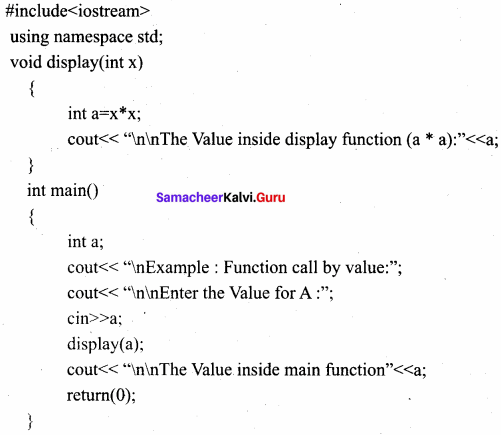Output:
Example : Function call by value
Enter the Value for A : 5
The Value inside display function (a * a) : 25
The Value inside main function 5

Question 2.
What is Recursion? Write a program to find GCD using recursion.
A function that calls itself is known as recursive function. And, this technique is known as recursion.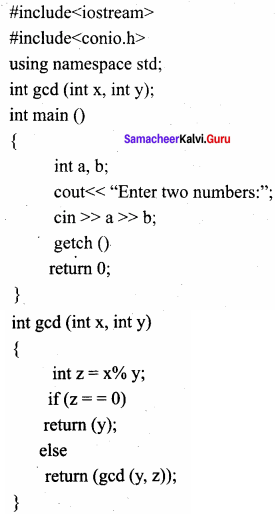Output:
Enter two numbers: 81 153
gcd : 9

Question 3.
What are the different forms of function return? Explain with example.
The return statement:
Returning from the function is done by using the return statement. The return statement stops execution and returns to the calling function. When a return statement is executed, the function is terminated immediately at that point. The return statement is used to return from a function. It is categorized as a jump statement because it terminates the execution of the function and transfer the control to the called statement.
Syntax:
return expression/variable;

Example : retum(a + b); retum(a);
return; // to terminate the function

The Returning values:
The functions that return no value is declared as void. The data type of a function is treated as int, if no data type is explicitly mentioned. For example,
For Example:
In both prototypes, the return value is int, because by default the return value of a function in C++ is of type int.

Returning Non – integer values:
A string can also be returned to a calling statement.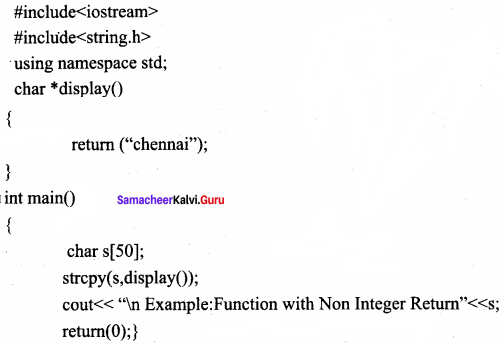Output:
Example: Function with Non-Integer Return Chennai

The Returning by reference:
#include
using namespace std;
int main( )
{

int n 1 = 150;
int &n 1 ref = n1;
cout << “\nThe Value of N1 = “<< n1 << “and n 1 Reference =”<< n 1 ref;
n 1 ref++;
cout << “\n After nl increased the Value of N1 =”<< n1;
cout << “and n 1 Reference = ”<< n 1 ref;
retum(0);

}
Output:
The Value of N1 = 150 and nl Reference =150
After n1 increased the Value of N1 = 151 and n1 Reference =151

Question 4.
Explain scope of variable with example.
A scope is a region or life of the variable. Scope refers to the accessibility of a variable. There are four types of scopes in C++. They are Local scope, Function scope, File scope and Class scope.

Local Scope:
A local variable is defined within a block. A block of code begins and ends with curly braces { }.
The scope of a local variable is the block in which it is defined.
A local variable cannot be accessed from outside the block of its declaration.
A local variable is created upon entry into its block and destroyed upon exit.

Example:
if (a > b)
{
int temp; //local to this if block//
temp = a;
a = b;
b = temp;
}

Function Scope:
The scope of variables declared within a function is extended to the function block, and all sub-blocks therein.
The life time of a function scope variable, is the life time of the function block. The scope of formal parameters is function scope.

Example:
{
int m=x+y; //’m’ declared within function add()// cout<<“\nThe Sum = “<<m;
}

File Scope:
A variable declared above all blocks and functions (including main ()) has the scope of a file. The life time of a file scope variable is the lifetime of a program.
The file scope variable is also called a global variable.

Example:
int file_var=20; //Declared within File// void add(int x, int y)
{
int m=x+y+file_var;
cout<<“\n The Sum = “<<m;
}

Question 5.
Write a program to accept any integer number and reverse it.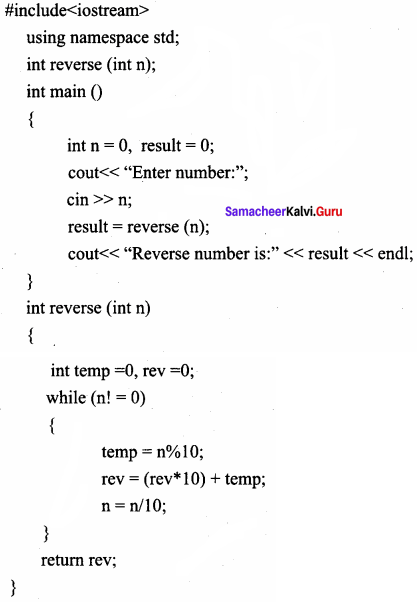Output:
Enter number : 1 2 3
Reverse number: 3 2 1

PART – 1

Question 1.
A large program can be split into small sub-programs called……………
a) Arrays
b) Functions
c) Structures
d) Unions
b) Functions

Question 2.
………………. is used to check whether the given character is an alphabet or not.
(a) isalnum()
(b) isalpha()
(c) isalph()
(d) isal()
(b) isalpha()

Question 3.
The functions which are available by default known as …………….. functions.
a) Built-in
b) User-defined
c) Either A or B
d) None of these
a) Built-in

Question 4.
………………. takes a null-terminated byte string source as its argument and returns its length.
(a) strcpy()
(b) strlen()
(c) strcmp()
(d) strcat()
(b) strlen()

Question 5.
Functions created by users is called ………………. functions.
a) Built-in
b) User-defined
c) Either A or B
d) None of these
b) User-defined

Question 6.
………………. is the name of the function.
(a) fact
(c) arguments
(d) None of these
(d) none of these

Question 7.
Complicated programs can be divided into manageable subprograms called functions using ………………
a) Divide and conquer
b) Reusability of code
c) Both A and B
d) All the above
a) Divide and conquer

Question 8.
Arguments are also called……………….
(a) variable
(b) constant
(c) function
(d) parameters
(d) parameters

Question 9.
……………… provide function prototype and definitions for library functions.
b) Pre-processor directive
c) Structures
d) None of these

Question 10.
Inline functions execute faster but require more ……………….
(a) variables
(b) pointers
(c) memory
(d) functions
(c) memory

Question 11.
………….. function will return 1 if the given character is an alphabet, and 0 otherwise 0.
a) isalnum( )
b) isalpha( )
c) isdigit( )
d) None of these
b) isalpha( )

Question 12.
………… function is used to check whether a given character is a digit or not.
a) isalnum( )
b) isalpha( )
c) isdigit( )
d) None of these
c) isdigit( )

Question 13.
……………. function will return 1 if the given character is a digit, and 0 otherwise.
a) isalnum( )
b) isalpha( )
c) isdigit( )
d) None of these
c) isdigit( )

Question 14.
………………. function is used to check whether a character is in lower case (small letter) or not.
a) islower( )
b) tolower( )
c) Both A and B
d) None of these
a) islower( )

Question 15.
……………. functions will return a non-zero value if the given character is a lower case alphabet, and 0 otherwise.
a) islower( )
b) tolower( )
c) toupper( )
d) isupper( )
a) islower( )

Question 16.
……………. function is used to check the given character is uppercase.
a) islower( )
b) tolower( )
c) toupper( )
d) isupper( )
d) isupper( )

Question 17.
……………. function will return 1 if the given character is an uppercase alphabet otherwise 0.
a) islower( )
b) tolower( )
c) toupper( )
d) isupper( )
d) isupper( )

Question 18.
………….. functions can be used to reduce the overheads like STACKS for small function definition.
a) Inline
b) Built-in
c) User-defined
d) None of these
a) Inline

Question 19.
……………. reduces the speed of program execution.
a) Array
b) Stacks
c) Structures
d) Unions
b) Stacks

Question 20.
What is the return type of the following function prototype? double add (int, int);
a) float
b) void
c) double
d) int
c) double

Question 21.
What is the return type of the following function prototype?
char *display();
a) float
b) string
c) double
d) char
b) string

Question 22.
A function that calls itself is known as ………….. function.
a) recursive
b) nested
c) invariant
d) variant
a) recursive

Question 23.
A function that calls itself using …………. technique.
a) recursive
b) variant
c) invariant
d) recursion
d) recursion

Question 24.
………………. is mandatory when a function is defined after the main() function.
a) Function prototype
b) Function parameters
c) Return statement
d) None of these
a) Function prototype

Question 25.
Scope refers to the accessibility of a ……………..
a) Function
b) Class
c) Variable
d) Constant
c) Variable

PART – 2

Question 1.
What is a user-defined function and built-in function?

• Built-in functions – Functions which are available in the C++ language standard library.
• User-defined functions – Functions created by users.

Question 2.
What are user-defined functions?
C++ also provides the facility to create new functions for a specific task as per user requirements. The name of the task and data required (arguments) are decided by the user and hence they are known as User-defined functions.

Question 3.
Write a note on divide and conquer.
Divide and Conquer:

• Complicated programs can be divided into manageable subprograms called functions.
• A programmer can focus on developing, debugging, and testing individual functions.
• Many programmers can work on different functions simultaneously.

Question 4.
What are the advantages of inline functions?

1. Inline functions execute faster but require more memory space.
2. Reduce the complexity of using STACKS.

Question 5.
What is the classification of a function?
Functions can be classified into two types,

• Pre-defined or Built-in or Library Functions
• User-defined Function.

Question 6.
What is the purpose of strupr( ) and strlwr( ) functions?

• The strupr( ) function is used to convert the given string into Uppercase letters.
• The strlwr( ) function is used to convert the given string into Lowercase letters.

PART – 3

Question 1.
WrIte about lsalnum( ) function with suitable example .
Isalnum( )
This function ¡s used to check whether a character is alphanumeric or not.
Syntax:
int isainum (char c)
This function returns a non-zero value if c is a digit or a letter, else it returns 0.

Example:
int r = isalnum’5′);
cout << isalnum’A’) <<‘\t'<<r;
The statements given below assign 0 to the variable n, since the given character is neither an alphabet nor a digit,
char c =‘\$‘;
int n = isalnum(c);
cout<<c;
Output
0
PROGRAM
#include <iostream>
#include <stdio.h>
#include <ctype.h>
using namespace std;
int main()
{
char ch;
int r;
cout<<“\n Type a Character :”;
ch = getcharQ;
r = isalnum(ch);
cout<<“\nThe Return Value of isalnum(ch) is :”<<r,
}
Output-1:
Type a Character :A
The Return Value of isa1num(ch) is
Output – 2:
Type a CharacterThe Return Value of isa1num(ch) is :0

Question 2.
Define library functions.
C++ provides a rich collection of functions ready to be used for various tasks. The tasks to be performed by each of these are already written, debugged, and compiled, their definitions alone are grouped and stored in files called header files. Such ready-to-use subprograms are called predefined functions or built-in functions or Library Functions.

Question 3.
tolower( )
This function is used to convert the given character into its lowercase. This function will return the lower case equivalent of the given character. If the given character itself is in lower case, the output will be the same.

Syntax:
char tolower(char c);

The following statement will assign the character constant ‘k’ to the variable c.
char c = tolower(‘K’);
The output of the statement given below will be ‘b’ itself.
cout <<tolower(‘b’);

Question 4.
Write a program using pow() and sin() function.
The pow() function returns base raised to the power of the exponent. The sin() function takes a single argument in radians.
#include
#include using namespace std;
int main ()
{

double base, exponent, result;
base = 5;
exponent = 4;
result = pow(base, exponent);
cout << “pow(“ << base << “A” << exponent <<”) =” << result;
double x = 25;
result = sin(x);
cout << “\nsin(“<< x <<”)=”<<result;
return 0;

}
Output:
pow (5^4) = 625
sin (25) = – 0.132352

Question 5.
How will you append (concatenate) strings?
strcat( ) function appends a copy of the character string pointed by the source to the end of the string pointed by the target. The strcat( ) function takes two arguments: target and source.
Example:
#include <string.h>
#include <iostream>
using namespace std;
int main()
{
char target = “Learning C++ is fun”;
char source = ” , easy and Very useful”;
strcat(target, source);
cout << target;
return 0;
}
Output
Learning C++ is fun, easy, and Very useful.

Question 6.
What is scope resolution operation?

1. The scope operator reveals the hidden scope of a variable. The scope resolution operator (::) is used for the following purposes.
2. To access a Global variable when there is a Local variable with the same name. An example using Scope Resolution Operator.

PART – 4
IV. Explain in Detail

Question 1.
Explain user-defined function with an example.
User-defined functions are created by the user. A function can optionally define input parameters that enable callers to pass arguments into the function. A function can also optionally return a value as output. Functions are useful for encapsulating common operations in a single reusable block, ideally with a name that clearly describes what the function does.

Function Definition:
In C++, a function must be defined before it is used anywhere in the program. The general syntax of a function definition is:
Return_Data_Type Function_name (parameter list)
{
Body of the function
}

• The Return_Data_Type is any valid data type of C++.
• The Function_name is a user-defined identifier.
• The parameter list, which is optional, is a list of parameters, i.e. a list of variables preceded by data types and separated by commas.
• The body of the function comprises C++ statements that are required to perform the intended task of this function.

Question 2.
Explain about Inline functions with a suitable program.
An inline function looks like a normal function in the source file but inserts the function’s code directly into the calling program. To make a function inline, one has to insert the keyword inline in the function header.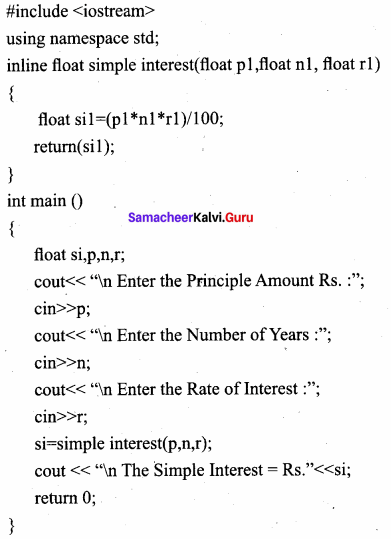Output:
Enter the Principle Amount Rs. :60000
Enter the Number of Years: 10
Enter the Rate of Interest: 5
The Simple Interest = Rs. 30000

Question 3.
C++ program can contain any number of functions. But, it must always have only one main() function to begin the program execution. We can write the definitions of functions in any order as we wish. We can define the main() function first and all other functions after that or we can define all the needed functions prior to main(). Like a variable declaration, a function must be declared before it is used in the program. The declaration statement may be given outside the main() function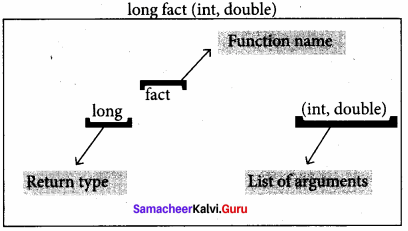The prototype above provides the following information to the compiler:

1. The return value of the function is of type long.
2. Fact is the name of the function.
3. The function is called with two arguments:
• The first argument is of the int data type.
• The second argument is of double data type, int display(int, int)//function prototype//

The above function prototype provides details about the return data type, the name of the function, and a list of formal parameters or arguments.

Question 4.
Explain call by reference with an example.
Call by reference or address method:
This method copies the address of the actual argument into the formal parameter. Since the address of the argument is passed, any change made in the formal parameter will be reflected back in the actual parameter.

PROGRAM:
#include <iostream>
using namespace std;
void display(int &x) //passing address of a//
{
x=x*x;
cout<<“\n\nThe Value inside display function (n1 x n1) :”< }
int main( )
{
intn1;
cout<<“\nEnter the Value for N1 cin>>n1;
cout<<“\nThe Value of N1 is inside main function Before passing : “<< n1; display(n1);
cout<<“\nThe Value of N1 is inside main function After passing (n1 x n1) : “<< n1; return(O);
}
Output:
Enter the Value for N1:45
The Value of N1 is inside main function Before passing: 45
The Value inside display function (n1 x n1) :2025
The Value of N1 is inside main function After passing (n1 x n1): 2025

The & symbol in the declaration of the parameter x means that the argument is a reference variable and hence the function will be called by passing reference. Hence when the argument num1 is passed to the display( ) function, the variable x gets the address of num1 so that the location will be shared.

In other words, the variables x and num1 refer to the same memory location. We use the name num1 in the main() function, and the name x in the display() function to refer to the same storage location. So, when we change the value of x, we are actually changing the value of num1.

Question 5.
Given data: Principal amount Rs. 50000

Number of years 5
Rate of interest 7

To find the simple interest of the above-mentioned given data. Write a C++ program using inline functions.
#include
using namespace std;
inline float simple interest(float p1, float n 1, float r 1)
{

float sil=(pl*nl*rl)/100;
retum(sil);

}
int main ()
{

float si,p,n,r;
cout << “\n Enter the Principle Amount Rs. :”; cin >> p;
cout << “\n Enter the Number of Years :”; cin >> n;
cout << “\n Enter the Rate of Interest :”; cin >> r;
si = simple interest(p, n, r);
cout << “\n The Simple Interest = Rs.” << si;
return 0;

}

Output:
Enter the Principle Amount Rs. 50000
Enter the Number of Years: 5
Enter the Rate of Interest: 7
The Simple Interest = Rs. 17500

## How to Prepare using Samacheer Kalvi 11th Computer Science Chapter 11 Functions Notes PDF?

Students must prepare for the upcoming exams from Samacheer Kalvi 11th Computer Science Chapter 11 Functions Notes PDF by following certain essential steps which are provided below.

• Use Samacheer Kalvi 11th Computer Science Chapter 11 Functions notes by paying attention to facts and ideas.
• Pay attention to the important topics
• Refer TN Board books as well as the books recommended.
• Correctly follow the notes to reduce the number of questions being answered in the exam incorrectly
• Highlight and explain the concepts in details.

## Frequently Asked Questions on Samacheer Kalvi 11th Computer Science Chapter 11 Functions Notes

#### How to use Samacheer Kalvi 11th Computer Science Chapter 11 Functions Notes for preparation??

Read TN Board thoroughly, make separate notes for points you forget, formulae, reactions, diagrams. Highlight important points in the book itself and make use of the space provided in the margin to jot down other important points on the same topic from different sources.

#### How to make notes for Samacheer Kalvi 11th Computer Science Chapter 11 Functions exam?

Read from hand-made notes prepared after understanding concepts, refrain from replicating from the textbook. Use highlighters for important points. Revise from these notes regularly and formulate your own tricks, shortcuts and mnemonics, mappings etc.
Share: